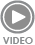# How to Reduce Jerk in Linear Motion Systems

Jerk is the derivative of acceleration, making it the third derivative of displacement.

The most common move profiles for linear motion systems are trapezoidal and triangular. In a trapezoidal move profile, the system accelerates from zero to its maximum speed, travels at that speed for a specified time (or distance), and then decelerates to zero. Conversely, the triangular move profile accelerates from zero to maximum speed and then immediately decelerates back to zero, with no constant velocity (i.e. all the move time is spent accelerating or decelerating).

But in reality, neither of these move profiles is particularly ideal for motion systems—especially those that require smooth travel, high positioning accuracy, or stability at the end of the move. This is because the process of accelerating and decelerating leads to a phenomenon known as jerk.

Just as acceleration is the rate of change (derivative) of velocity, jerk is the rate of change of acceleration. In other words, jerk is the rate at which acceleration is increasing or decreasing. Jerk is generally undesirable because it creates—you guessed it—abrupt, jerky motion. In industrial applications such as machine tools, SCARA robots, and dispensing systems, a rapid change in acceleration—i.e. jerk—causes the system to vibrate. The higher the jerk, the stronger the vibrations. And vibrations decrease positioning accuracy while increasing settling time.

The way to avoid jerk is to reduce the rate of acceleration or deceleration. In motion control systems, this is done by using an S-curve motion profile, instead of the “jerky” trapezoidal profile. In a trapezoidal move profile, acceleration occurs instantly (at least in theory) and jerk is infinite. To reduce the amount of jerk generated during the move, the transitions at the beginning and end of acceleration and deceleration are smoothed into an “S” shape. The resulting profile is referred to as an S-curve move profile.

If we plot the acceleration profile for a trapezoidal move (see above), we’ll see that it is a step function—that is, acceleration goes from zero to its maximum instantaneously, and deceleration goes from maximum to zero instantaneously. In an S-curve move, the acceleration profile becomes trapezoidal in shape, and acceleration and deceleration occur in a smooth manner, rather than instantly and abruptly.

The S-curve profile is based on a third-order system, making the motion equations for acceleration, velocity, and distance (displacement) more complex than those for trapezoidal move profiles.

The tradeoff of using an S-curve versus a trapezoidal move profile is that the overall time for the move is longer with an S-curve profile. This is because ramping acceleration (and deceleration) takes longer than the instantaneous acceleration of a trapezoidal move. However, the time advantage gained by using a trapezoidal move profile may be negated by a longer settling time, due to vibrations induced by high levels of jerk. And because jerk puts extensive strain on mechanical components, even if a trapezoidal move is used as the basis, some amount of smoothing is typically applied to the acceleration and deceleration phases, making the move profile more S-shaped.Details

• Chengdu, Sichuan, China
• FUYU Technology